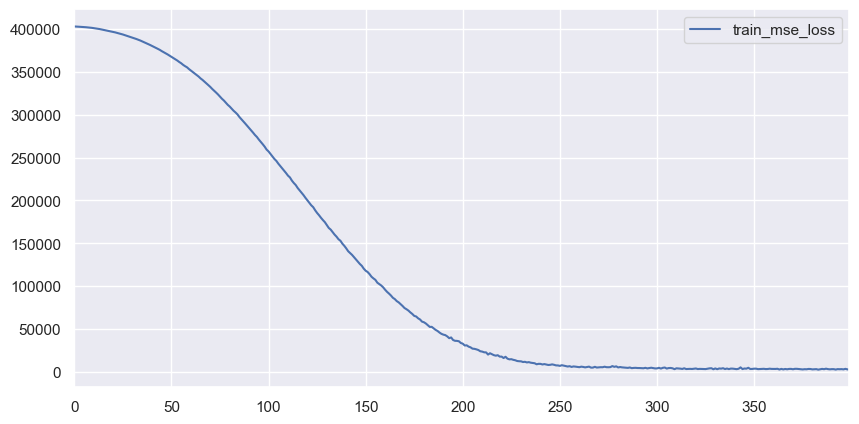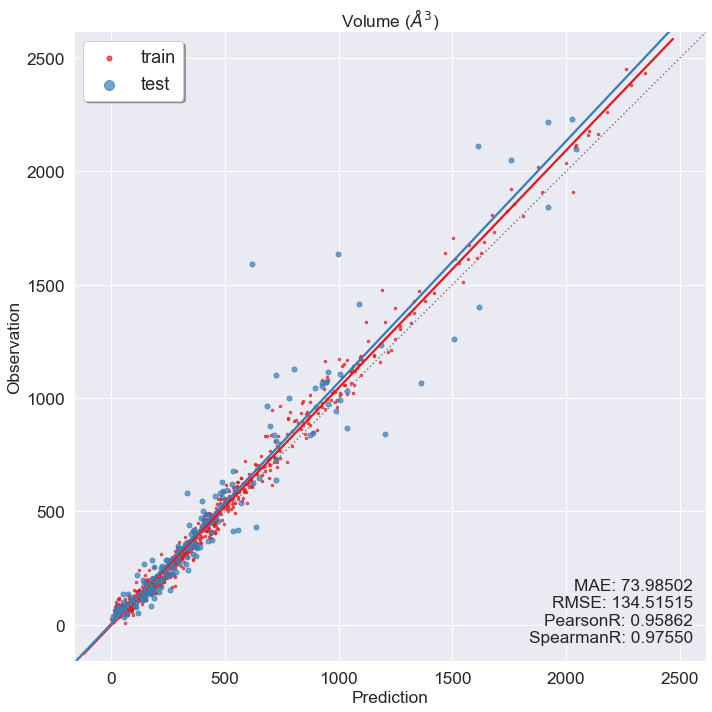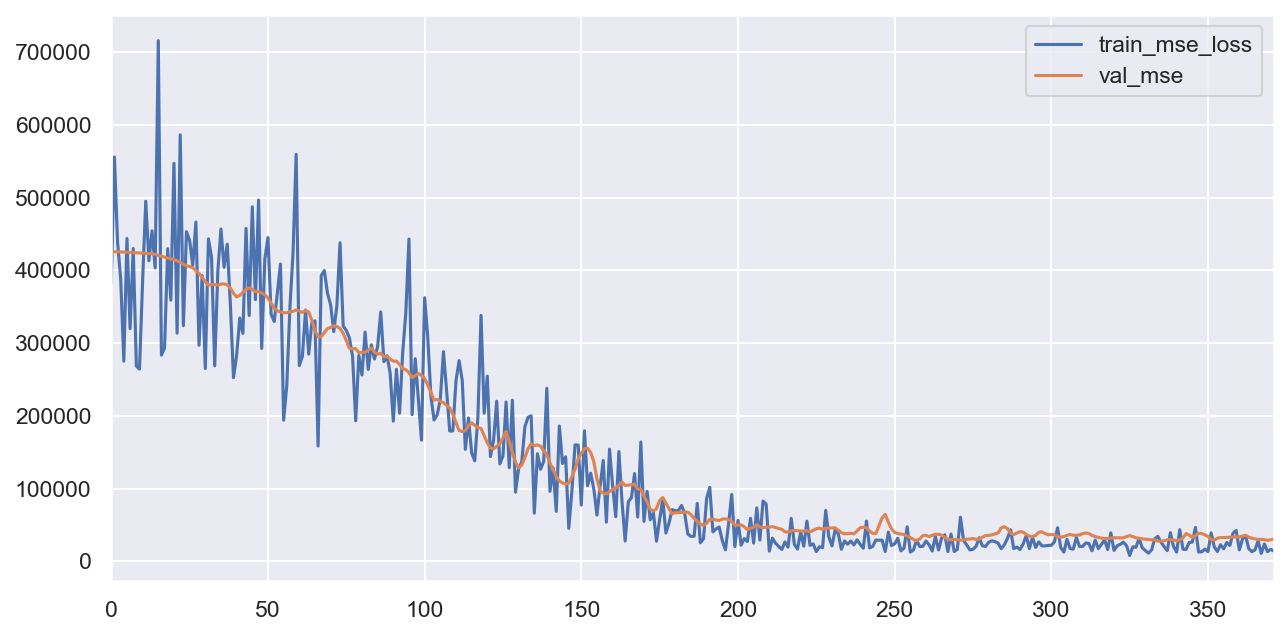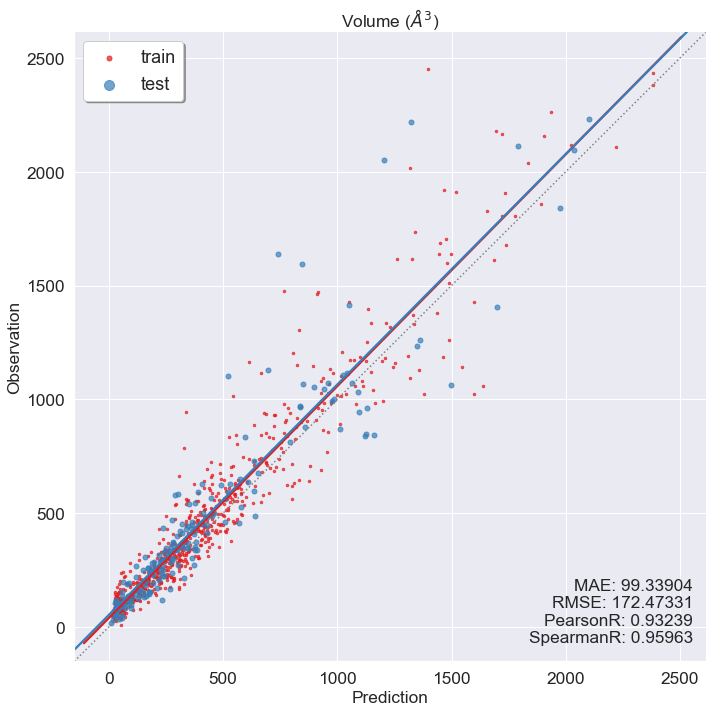# Random NN models

This tutorial shows how to build neural network models. We will learn how to calculate compositional descriptors using xenonpy.descriptor.Compositions calculator and train our model using xenonpy.model.training modules.

In this tutorial, we will use some inorganic sample data from materials project. If you don’t have it, please see https://github.com/yoshida-lab/XenonPy/blob/master/samples/build_sample_data.ipynb.

## useful functions

Running the following cell will load some commonly used packages, such as numpy, pandas, and so on. It will also import some in-house functions used in this tutorial. See samples/tools.ipynb to check what will be imported.

:

%run tools.ipynb


### Sequential linear model

We will use xenonpy.model.SequentialLinear to build a sequential linear model. The basic layer in SequentialLinear model is xenonpy.model.LinearLayer

:

from xenonpy.model import SequentialLinear, LinearLayer
SequentialLinear?

Init signature:
SequentialLinear(
in_features: int,
out_features: int,
bias: bool = True,
*,
h_neurons: Union[Tuple[float, ...], Tuple[int, ...]] = (),
h_bias: Union[bool, Tuple[bool, ...]] = True,
h_dropouts: Union[float, Tuple[float, ...]] = 0.1,
h_normalizers: Union[float, NoneType, Tuple[Union[float, NoneType], ...]] = 0.1,
h_activation_funcs: Union[Callable, NoneType, Tuple[Union[Callable, NoneType], ...]] = ReLU(),
)
Docstring:
Sequential model with linear layers and configurable other hype-parameters.
e.g. dropout, hidden layers
Init docstring:
Parameters
----------
in_features
Size of input.
out_features
Size of output.
bias
Enable bias in input layer.
h_neurons
Number of neurons in hidden layers.
Can be a tuple of floats. In that case,
all these numbers will be used to calculate the neuron numbers.
e.g. (0.5, 0.4, ...) will be expanded as (in_features * 0.5, in_features * 0.4, ...)
h_bias
bias in hidden layers.
h_dropouts
Probabilities of dropout in hidden layers.
h_normalizers
Momentum of batched normalizers in hidden layers.
h_activation_funcs
Activation functions in hidden layers.
File:           ~/projects/XenonPy/xenonpy/model/sequential.py
Type:           type
Subclasses:


:

LinearLayer?

Init signature:
LinearLayer(
in_features: int,
out_features: int,
bias: bool = True,
*,
dropout: float = 0.0,
activation_func: Callable = ReLU(),
normalizer: Union[float, NoneType] = 0.1,
)
Docstring:
Base NN layer. This is a wrap around PyTorch.
See here for details: http://pytorch.org/docs/master/nn.html#
Init docstring:
Parameters
----------
in_features:
Size of each input sample.
out_features:
Size of each output sample
dropout: float
Probability of an element to be zeroed. Default: 0.5
activation_func: func
Activation function.
normalizer: func
Normalization layers
File:           ~/projects/XenonPy/xenonpy/model/sequential.py
Type:           type
Subclasses:



Following the official suggestion from PyTorch, SequentialLinear is a python class that inherit the torch.nn.Module class. Users can specify the hyperparameters to get a fully customized model object. For example:

:

model = SequentialLinear(290, 1, h_neurons=(0.8, 0.7, 0.6))
model

:

SequentialLinear(
(layer_0): LinearLayer(
(linear): Linear(in_features=290, out_features=232, bias=True)
(dropout): Dropout(p=0.1)
(normalizer): BatchNorm1d(232, eps=0.1, momentum=0.1, affine=True, track_running_stats=True)
(activation): ReLU()
)
(layer_1): LinearLayer(
(linear): Linear(in_features=232, out_features=203, bias=True)
(dropout): Dropout(p=0.1)
(normalizer): BatchNorm1d(203, eps=0.1, momentum=0.1, affine=True, track_running_stats=True)
(activation): ReLU()
)
(layer_2): LinearLayer(
(linear): Linear(in_features=203, out_features=174, bias=True)
(dropout): Dropout(p=0.1)
(normalizer): BatchNorm1d(174, eps=0.1, momentum=0.1, affine=True, track_running_stats=True)
(activation): ReLU()
)
(output): Linear(in_features=174, out_features=1, bias=True)
)


## fully randomized model generation

Process of random model generation:

1. using a random parameter generator to generate a set of parameter.

2. using the generated parameter set to setup a model object.

3. loop step 1 and 2 as many times as needed.

We provided a general parameter generator xenonpy.utils.ParameterGenerator to do all the 3 steps for any callable object.

:

from xenonpy.utils import ParameterGenerator
ParameterGenerator?

Init signature:
ParameterGenerator(
seed: Union[int, NoneType] = None,
**kwargs: Union[Any, Sequence, Callable, Dict],
)
Docstring:      Generator for parameter set generating.
Init docstring:
Parameters
----------
seed
Numpy random seed.
kwargs
Parameter candidate.
File:           ~/projects/XenonPy/xenonpy/utils/parameter_gen.py
Type:           type
Subclasses:



Calling an instance of ParameterGenerator will return a generator. This generator can randomly select parameters from parameter candidates and yield them as a dict. This is what we want in step 1.

:

from math import ceil
from random import uniform

generator = ParameterGenerator(
in_features=290,
out_features=1,
h_neurons=dict(
data=[ceil(uniform(0.1, 1.2) * 290) for _ in range(100)],
repeat=(2, 3)
)
)


Because generator is a generator, for ... in ... statement can be applied. For example, we can use for parameters in generator(num_of_models) to loop all these generated parameter sets.

:

for parameters in generator(num=10):
print(parameters)

{'in_features': 290, 'out_features': 1, 'h_neurons': (70, 109)}
{'in_features': 290, 'out_features': 1, 'h_neurons': (135, 45)}
{'in_features': 290, 'out_features': 1, 'h_neurons': (216, 261, 79)}
{'in_features': 290, 'out_features': 1, 'h_neurons': (111, 88, 49)}
{'in_features': 290, 'out_features': 1, 'h_neurons': (216, 79, 47)}
{'in_features': 290, 'out_features': 1, 'h_neurons': (161, 45)}
{'in_features': 290, 'out_features': 1, 'h_neurons': (193, 161, 78)}
{'in_features': 290, 'out_features': 1, 'h_neurons': (90, 36, 234)}
{'in_features': 290, 'out_features': 1, 'h_neurons': (230, 83, 295)}
{'in_features': 290, 'out_features': 1, 'h_neurons': (171, 247)}


For step 2, we can give a model class to the factory parameter as a factory function. If factory parameter is given, generator will feed generated parameters to the factory function automatically and yield the result as a second return in each loop. For example:

:

for parameters, model in generator(2, factory=SequentialLinear):
print('parameters: ', parameters)
print(model, '\n')

parameters:  {'in_features': 290, 'out_features': 1, 'h_neurons': (182, 335, 204)}
SequentialLinear(
(layer_0): LinearLayer(
(linear): Linear(in_features=290, out_features=182, bias=True)
(dropout): Dropout(p=0.1)
(normalizer): BatchNorm1d(182, eps=0.1, momentum=0.1, affine=True, track_running_stats=True)
(activation): ReLU()
)
(layer_1): LinearLayer(
(linear): Linear(in_features=182, out_features=335, bias=True)
(dropout): Dropout(p=0.1)
(normalizer): BatchNorm1d(335, eps=0.1, momentum=0.1, affine=True, track_running_stats=True)
(activation): ReLU()
)
(layer_2): LinearLayer(
(linear): Linear(in_features=335, out_features=204, bias=True)
(dropout): Dropout(p=0.1)
(normalizer): BatchNorm1d(204, eps=0.1, momentum=0.1, affine=True, track_running_stats=True)
(activation): ReLU()
)
(output): Linear(in_features=204, out_features=1, bias=True)
)

parameters:  {'in_features': 290, 'out_features': 1, 'h_neurons': (108, 255)}
SequentialLinear(
(layer_0): LinearLayer(
(linear): Linear(in_features=290, out_features=108, bias=True)
(dropout): Dropout(p=0.1)
(normalizer): BatchNorm1d(108, eps=0.1, momentum=0.1, affine=True, track_running_stats=True)
(activation): ReLU()
)
(layer_1): LinearLayer(
(linear): Linear(in_features=108, out_features=255, bias=True)
(dropout): Dropout(p=0.1)
(normalizer): BatchNorm1d(255, eps=0.1, momentum=0.1, affine=True, track_running_stats=True)
(activation): ReLU()
)
(output): Linear(in_features=255, out_features=1, bias=True)
)



We also enable users to generate parameters in a functional way by given a function as candidate parameters. In this case, the function accept an int number as vector length and a vector of generated parameters. For example, generate a n length vector with float numbers sorted in ascending.

:

generator = ParameterGenerator(
in_features=290,
out_features=1,
h_neurons=dict(
data=lambda n: sorted(np.random.uniform(0.2, 0.8, size=n), reverse=True),
repeat=(2, 3)
)
)

:

for parameters, model in generator(2, factory=SequentialLinear):
print('parameters: ', parameters)
print(model, '\n')

parameters:  {'in_features': 290, 'out_features': 1, 'h_neurons': (0.7954011465554711, 0.6993610045591458)}
SequentialLinear(
(layer_0): LinearLayer(
(linear): Linear(in_features=290, out_features=231, bias=True)
(dropout): Dropout(p=0.1)
(normalizer): BatchNorm1d(231, eps=0.1, momentum=0.1, affine=True, track_running_stats=True)
(activation): ReLU()
)
(layer_1): LinearLayer(
(linear): Linear(in_features=231, out_features=203, bias=True)
(dropout): Dropout(p=0.1)
(normalizer): BatchNorm1d(203, eps=0.1, momentum=0.1, affine=True, track_running_stats=True)
(activation): ReLU()
)
(output): Linear(in_features=203, out_features=1, bias=True)
)

parameters:  {'in_features': 290, 'out_features': 1, 'h_neurons': (0.798810246990777, 0.38584535382368323, 0.29569841893337045)}
SequentialLinear(
(layer_0): LinearLayer(
(linear): Linear(in_features=290, out_features=232, bias=True)
(dropout): Dropout(p=0.1)
(normalizer): BatchNorm1d(232, eps=0.1, momentum=0.1, affine=True, track_running_stats=True)
(activation): ReLU()
)
(layer_1): LinearLayer(
(linear): Linear(in_features=232, out_features=112, bias=True)
(dropout): Dropout(p=0.1)
(normalizer): BatchNorm1d(112, eps=0.1, momentum=0.1, affine=True, track_running_stats=True)
(activation): ReLU()
)
(layer_2): LinearLayer(
(linear): Linear(in_features=112, out_features=86, bias=True)
(dropout): Dropout(p=0.1)
(normalizer): BatchNorm1d(86, eps=0.1, momentum=0.1, affine=True, track_running_stats=True)
(activation): ReLU()
)
(output): Linear(in_features=86, out_features=1, bias=True)
)



### Model training

We provide a general and extendable model training system for neural network models. By customizing the extensions, users can fully control their model training process, and save the training results following the XenonPy.MDL format automatically.

All these modules and extensions are under the xenonpy.model.training.

:

import torch
import matplotlib.pyplot as plt
from pymatgen import Structure

from xenonpy.model.training import Trainer, SGD, MSELoss, Adam, ReduceLROnPlateau, ExponentialLR, ClipValue

from xenonpy.datatools import preset, Splitter
from xenonpy.descriptor import Compositions


#### prepare training/testing data

If you followed the tutorial in https://github.com/yoshida-lab/XenonPy/blob/master/samples/build_sample_data.ipynb, the sample data should be save at ~/.xenonpy/userdata with name mp_samples.pd.xz. You can use pd.read_pickle to load this file but we suggest that you use our xenonpy.datatools.preset module.

We chose volume as the example to demonstrate how to use our training system. We only use the data which volume are smaller than 2,500 to avoid the disparate data. we select data in pandas.DataFrame and calculate the descriptors using xenonpy.descriptor.Compositions calculator. It is noticed that the input for a neuron network model in pytorch must has shape (N x M x …), which mean if the input is a 1-D vector, it should be reshaped to 2-D, e.g. (N) -> (N x 1).

:

# if you have not have the samples data
# preset.build('mp_samples', api_key=<your materials project api key>)

from xenonpy.datatools import preset

data = preset.mp_samples

:

band_gap composition density e_above_hull efermi elements final_energy_per_atom formation_energy_per_atom pretty_formula structure volume
mp-1008807 0.0000 {'Rb': 1.0, 'Cu': 1.0, 'O': 1.0} 4.784634 0.996372 1.100617 [Rb, Cu, O] -3.302762 -0.186408 RbCuO [[-3.05935361 -3.05935361 -3.05935361] Rb, [0.... 57.268924
mp-1009640 0.0000 {'Pr': 1.0, 'N': 1.0} 8.145777 0.759393 5.213442 [Pr, N] -7.082624 -0.714336 PrN [[0. 0. 0.] Pr, [1.57925232 1.57925232 1.58276... 31.579717
mp-1016825 0.7745 {'Hf': 1.0, 'Mg': 1.0, 'O': 3.0} 6.165888 0.589550 2.424570 [Hf, Mg, O] -7.911723 -3.060060 HfMgO3 [[2.03622802 2.03622802 2.03622802] Hf, [0. 0.... 67.541269

In case of the system did not automatically download some descriptor data in XenonPy, please run the following code to sync the data.

:

from xenonpy.datatools import preset
preset.sync('elements')
preset.sync('elements_completed')

fetching dataset elements from https://github.com/yoshida-lab/dataset/releases/download/v0.1.3/elements.pd.xz.
fetching dataset elements_completed from https://github.com/yoshida-lab/dataset/releases/download/v0.1.3/elements_completed.pd.xz.

:

prop = data[data.volume <= 2500]['volume'].to_frame()  # reshape to 2-D
desc = Compositions(featurizers='classic').transform(data.loc[prop.index]['composition'])


:

mp-1008807 24.666667 174.067140 209.333333 25.666667 55.004267 1297.063333 72.868680 1646.90 139.333333 128.333333 ... 1.0 2.0 0.360 0.02658 152.0 150.0 182.0 349.5 317.5 0.802
mp-1009640 33.000000 137.000000 232.500000 19.050000 77.457330 1931.200000 43.182441 1892.85 137.000000 123.500000 ... 2.0 2.0 0.192 0.02583 155.0 166.0 193.0 360.6 333.6 1.100
mp-1016825 21.600000 153.120852 203.400000 13.920000 50.158400 1420.714000 76.663625 343.82 102.800000 96.000000 ... 2.0 2.0 0.146 0.02658 152.0 150.0 182.0 302.1 317.5 0.802

3 rows × 290 columns

:

volume
mp-1008807 57.268924
mp-1009640 31.579717
mp-1016825 67.541269
:

from xenonpy.datatools import preset


Use the xenonpy.datatools.Splitter to split data into training and test sets.

:

Splitter?

Init signature:
Splitter(
size: int,
*,
test_size: Union[float, int] = 0.2,
k_fold: Union[int, Iterable, NoneType] = None,
random_state: Union[int, NoneType] = None,
shuffle: bool = True,
)
Docstring:      Data splitter for train and test
Init docstring:
Parameters
----------
size
Total sample size.
All data must have same length of their first dim,
test_size
If float, should be between 0.0 and 1.0 and represent the proportion
of the dataset to include in the test split. If int, represents the
absolute number of test samples. Can be 0 if cv is None.
In this case, :meth:~Splitter.cv will yield a tuple only contains training and validation
on each step. By default, the value is set to 0.2.
k_fold
Number of k-folds.
If int, Must be at least 2.
If Iterable, it should provide label for each element which will be used for group cv.
In this case, the input of :meth:~Splitter.cv must be a :class:pandas.DataFrame object.
Default value is None to specify no cv.
random_state
If int, random_state is the seed used by the random number generator;
Default is None.
shuffle
Whether or not to shuffle the data before splitting.
File:           ~/projects/XenonPy/xenonpy/datatools/splitter.py
Type:           type
Subclasses:


:

sp = Splitter(prop.shape)
x_train, x_val, y_train, y_val = sp.split(desc, prop)

x_train.shape
y_train.shape
x_val.shape
y_val.shape

:

(738, 290)

:

(738, 1)

:

(185, 290)

:

(185, 1)


#### model training

Model training in pytorch is very flexible. The official document explains the concept with examples. In short, training a neuron network model includes: 1. calculating loss of training data for the model. 2. executing the backpropagation to update the weights between each neuron. 3. looping over step 1 and 2 until convergence.

In step 1, a calculator, often called loss function, is needed to calculate the loss. In step 2, an optimizer will be used. xenonpy.model.training.Trainer covers step 3.

Here is how you will do it in XenonPy:

:

model = SequentialLinear(290, 1, h_neurons=(0.8, 0.6, 0.4, 0.2))
model

:

SequentialLinear(
(layer_0): LinearLayer(
(linear): Linear(in_features=290, out_features=232, bias=True)
(dropout): Dropout(p=0.1)
(normalizer): BatchNorm1d(232, eps=0.1, momentum=0.1, affine=True, track_running_stats=True)
(activation): ReLU()
)
(layer_1): LinearLayer(
(linear): Linear(in_features=232, out_features=174, bias=True)
(dropout): Dropout(p=0.1)
(normalizer): BatchNorm1d(174, eps=0.1, momentum=0.1, affine=True, track_running_stats=True)
(activation): ReLU()
)
(layer_2): LinearLayer(
(linear): Linear(in_features=174, out_features=116, bias=True)
(dropout): Dropout(p=0.1)
(normalizer): BatchNorm1d(116, eps=0.1, momentum=0.1, affine=True, track_running_stats=True)
(activation): ReLU()
)
(layer_3): LinearLayer(
(linear): Linear(in_features=116, out_features=58, bias=True)
(dropout): Dropout(p=0.1)
(normalizer): BatchNorm1d(58, eps=0.1, momentum=0.1, affine=True, track_running_stats=True)
(activation): ReLU()
)
(output): Linear(in_features=58, out_features=1, bias=True)
)

:

trainer = Trainer(
model=model,
loss_func=MSELoss()
)
trainer

:

Trainer(clip_grad=None, cuda=None, epochs=200, loss_func=MSELoss(),
lr_scheduler=None,
model=SequentialLinear(
(layer_0): LinearLayer(
(linear): Linear(in_features=290, out_features=232, bias=True)
(dropout): Dropout(p=0.1)
(normalizer): BatchNorm1d(232, eps=0.1, momentum=0.1, affine=True, track_running_stats=True)
(activation): ReLU()
)
(layer_1): LinearLayer(
(linear): Li...
(linear): Linear(in_features=116, out_features=58, bias=True)
(dropout): Dropout(p=0.1)
(normalizer): BatchNorm1d(58, eps=0.1, momentum=0.1, affine=True, track_running_stats=True)
(activation): ReLU()
)
(output): Linear(in_features=58, out_features=1, bias=True)
),
non_blocking=False,
Parameter Group 0
betas: (0.9, 0.999)
eps: 1e-08
lr: 0.01
weight_decay: 0
))

:

trainer.fit(
x_train=torch.tensor(x_train.values, dtype=torch.float),
y_train=torch.tensor(y_train.values, dtype=torch.float),
epochs=400
)

Training: 100%|██████████| 400/400 [00:06<00:00, 60.86it/s]

:

_, ax = plt.subplots(figsize=(10, 5), dpi=100)
trainer.training_info.tail(3)
trainer.training_info.plot(y=['train_mse_loss'], ax=ax)

:

total_iters i_epoch i_batch train_mse_loss
397 397 398 1 3684.717041
398 398 399 1 3058.761719
399 399 400 1 3039.683350
:

<matplotlib.axes._subplots.AxesSubplot at 0x1a2ce256d0>:

y_pred = trainer.predict(x_in=torch.tensor(x_val.values, dtype=torch.float)).detach().numpy().flatten()
y_true = y_val.values.flatten()

y_fit_pred = trainer.predict(x_in=torch.tensor(x_train.values, dtype=torch.float)).detach().numpy().flatten()
y_fit_true = y_train.values.flatten()

draw(y_true, y_pred, y_fit_true, y_fit_pred, prop_name='Volume ($\AA^3$)')

Missing directory and/or file name information!You can see that although trainer can keep training info automatically for us, we still need to convert DataFrame to torch.Tensor and convert dtype from np.double to torch.float during training, and eventually convert them back during prediction ourselves. Also, overfitting could be observed in the prediction vs observation plot. One possible caused is using all training data in each epoch of the training process. We can use a technique called mini-batch to avoid overfitting, but that will require more coding.

To skip these tedious coding steps, we provide an extension system.

First, we use ArrayDataset to wrap our data, and use torch.utils.data.DataLoader to a build mini-batch loader.

:

from xenonpy.model.training.dataset import ArrayDataset

:

train_dataset = DataLoader(ArrayDataset(x_train, y_train), shuffle=True, batch_size=100)


Second, we use TensorConverter extension to automatically convert data between numpy and torch.Tensor. Additionally, we want to trace some stopping criterion and use early stopping when these criterion stop improving. This can be done by using Validator.

At last, to save our model for latter use, just add Persist to the trainer and saving will be done in slient.

:

from xenonpy.model.training.extension import Validator, TensorConverter, Persist
from xenonpy.model.training.dataset import ArrayDataset
from xenonpy.model.utils import regression_metrics


We use trainer.extend method to stack up extensions. Note that extensions will be executed in the order of insertion, so TensorConverter should always go first and Persist be the last.

:

trainer = Trainer(
loss_func=MSELoss(),
).extend(
TensorConverter(),
Validator(metrics_func=regression_metrics, early_stopping=30, trace_order=5, mae=0.0, pearsonr=1.0),
)

:

Persist?

Init signature:
Persist(
path: Union[pathlib.Path, str] = '.',
*,
model_class: Callable = None,
model_params: Union[tuple, dict, <built-in function any>] = None,
increment=False,
sync_training_step=False,
**describe: Any,
)
Docstring:      Trainer extension for data persistence
Init docstring:
Parameters
----------
path
Path for model saving.
model_class
A factory function for model reconstructing.
In most case this is the model class inherits from :class:torch.nn.Module
model_params
The parameters for model reconstructing.
This can be anything but in general this is a dict which can be used as kwargs parameters.
increment
If True, dir name of path will be decorated with a auto increment number,
e.g. use model_dir@1 for model_dir.
sync_training_step
If True, will save trainer.training_info at each iteration.
Default is False, only save trainer.training_info at each epoch.
describe:
Any other information to describe this model.
These information will be saved under model dir by name describe.pkl.z.
File:           ~/projects/XenonPy/xenonpy/model/training/extension/persist.py
Type:           type
Subclasses:



Persist need a path to save model. For convenience, we prepare the path name by concatenating the number of neurons in a model.

:

def make_name(model):
name = []
for n, m in model.named_children():
if 'layer_' in n:
name.append(str(m.linear.in_features))
else:
name.append(str(m.in_features))
name.append(str(m.out_features))
return '-'.join(name)

:

model = SequentialLinear(290, 1, h_neurons=(0.8, 0.6, 0.4, 0.2))
model

model_name = make_name(model)
persist = Persist(
f'trained_models/{model_name}',
# -^- required -^-

# -v- optional -v-
increment=False,
sync_training_step=True,
author='Chang Liu',
email='liu.chang.1865@gmail.com',
dataset='materials project',
)
_ = trainer.extend(persist)
trainer.reset(to=model)

trainer.fit(training_dataset=train_dataset, validation_dataset=val_dataset, epochs=400)
persist(splitter=sp, data_indices=prop.index.tolist())  # <-- calling of this method only after the model training

:

SequentialLinear(
(layer_0): LinearLayer(
(linear): Linear(in_features=290, out_features=232, bias=True)
(dropout): Dropout(p=0.1)
(normalizer): BatchNorm1d(232, eps=0.1, momentum=0.1, affine=True, track_running_stats=True)
(activation): ReLU()
)
(layer_1): LinearLayer(
(linear): Linear(in_features=232, out_features=174, bias=True)
(dropout): Dropout(p=0.1)
(normalizer): BatchNorm1d(174, eps=0.1, momentum=0.1, affine=True, track_running_stats=True)
(activation): ReLU()
)
(layer_2): LinearLayer(
(linear): Linear(in_features=174, out_features=116, bias=True)
(dropout): Dropout(p=0.1)
(normalizer): BatchNorm1d(116, eps=0.1, momentum=0.1, affine=True, track_running_stats=True)
(activation): ReLU()
)
(layer_3): LinearLayer(
(linear): Linear(in_features=116, out_features=58, bias=True)
(dropout): Dropout(p=0.1)
(normalizer): BatchNorm1d(58, eps=0.1, momentum=0.1, affine=True, track_running_stats=True)
(activation): ReLU()
)
(output): Linear(in_features=58, out_features=1, bias=True)
)

Training:  12%|█▏        | 47/400 [00:15<02:06,  2.78it/s]

Early stopping is applied: no improvement for ['mae', 'pearsonr'] since the last 31 iterations, finish training at iteration 372



:

_, ax = plt.subplots(figsize=(10, 5), dpi=150)
trainer.training_info.plot(y=['train_mse_loss', 'val_mse'], ax=ax)

:

<matplotlib.axes._subplots.AxesSubplot at 0x1a2d100e10>:

y_pred, y_true = trainer.predict(dataset=val_dataset, checkpoint='pearsonr_1')
y_fit_pred, y_fit_true = trainer.predict(dataset=train_dataset, checkpoint='pearsonr_1')
draw(y_true, y_pred, y_fit_true, y_fit_pred, prop_name='Volume ($\AA^3$)')

Missing directory and/or file name information!### Combine random model generating and training

:

generator = ParameterGenerator(
in_features=290,
out_features=1,
h_neurons=dict(
data=lambda n: sorted(np.random.uniform(0.2, 0.8, size=n), reverse=True),
repeat=(3, 4)
)
)

:

for paras, model in generator(num=2, factory=SequentialLinear):
print(model)
model_name = make_name(model)
persist = Persist(
f'trained_models/{model_name}',
# -^- required -^-

# -v- optional -v-
increment=False,
sync_training_step=True,
model_class=SequentialLinear,
model_params=paras,
author='Chang Liu',
email='liu.chang.1865@gmail.com',
dataset='materials project',
)
_ = trainer.extend(persist)
trainer.reset(to=model)

trainer.fit(training_dataset=train_dataset, validation_dataset=val_dataset, epochs=400)
persist(splitter=sp, data_indices=prop.index.tolist())  # <-- calling of this method only after the model training

Training:   0%|          | 0/400 [00:00<?, ?it/s]

SequentialLinear(
(layer_0): LinearLayer(
(linear): Linear(in_features=290, out_features=195, bias=True)
(dropout): Dropout(p=0.1)
(normalizer): BatchNorm1d(195, eps=0.1, momentum=0.1, affine=True, track_running_stats=True)
(activation): ReLU()
)
(layer_1): LinearLayer(
(linear): Linear(in_features=195, out_features=141, bias=True)
(dropout): Dropout(p=0.1)
(normalizer): BatchNorm1d(141, eps=0.1, momentum=0.1, affine=True, track_running_stats=True)
(activation): ReLU()
)
(layer_2): LinearLayer(
(linear): Linear(in_features=141, out_features=131, bias=True)
(dropout): Dropout(p=0.1)
(normalizer): BatchNorm1d(131, eps=0.1, momentum=0.1, affine=True, track_running_stats=True)
(activation): ReLU()
)
(layer_3): LinearLayer(
(linear): Linear(in_features=131, out_features=61, bias=True)
(dropout): Dropout(p=0.1)
(normalizer): BatchNorm1d(61, eps=0.1, momentum=0.1, affine=True, track_running_stats=True)
(activation): ReLU()
)
(output): Linear(in_features=61, out_features=1, bias=True)
)

Training:  12%|█▏        | 48/400 [00:15<02:06,  2.79it/s]
Training:   0%|          | 0/400 [00:00<?, ?it/s]

Early stopping is applied: no improvement for ['mae', 'pearsonr'] since the last 31 iterations, finish training at iteration 380
SequentialLinear(
(layer_0): LinearLayer(
(linear): Linear(in_features=290, out_features=214, bias=True)
(dropout): Dropout(p=0.1)
(normalizer): BatchNorm1d(214, eps=0.1, momentum=0.1, affine=True, track_running_stats=True)
(activation): ReLU()
)
(layer_1): LinearLayer(
(linear): Linear(in_features=214, out_features=181, bias=True)
(dropout): Dropout(p=0.1)
(normalizer): BatchNorm1d(181, eps=0.1, momentum=0.1, affine=True, track_running_stats=True)
(activation): ReLU()
)
(layer_2): LinearLayer(
(linear): Linear(in_features=181, out_features=141, bias=True)
(dropout): Dropout(p=0.1)
(normalizer): BatchNorm1d(141, eps=0.1, momentum=0.1, affine=True, track_running_stats=True)
(activation): ReLU()
)
(layer_3): LinearLayer(
(linear): Linear(in_features=141, out_features=130, bias=True)
(dropout): Dropout(p=0.1)
(normalizer): BatchNorm1d(130, eps=0.1, momentum=0.1, affine=True, track_running_stats=True)
(activation): ReLU()
)
(output): Linear(in_features=130, out_features=1, bias=True)
)

Training:  10%|█         | 42/400 [00:14<02:18,  2.59it/s]

Early stopping is applied: no improvement for ['mae', 'pearsonr'] since the last 31 iterations, finish training at iteration 333



[ ]: